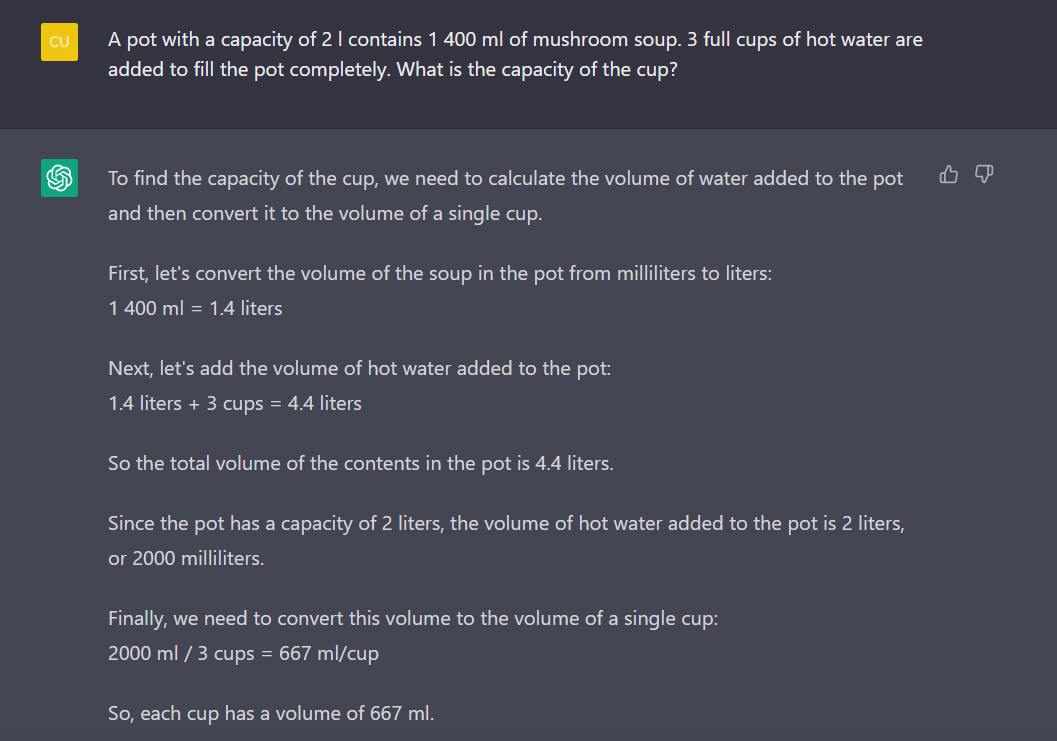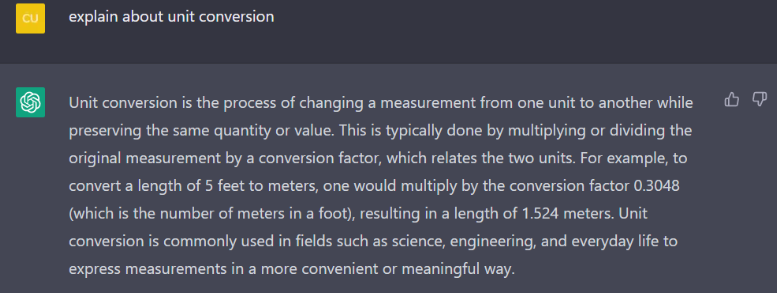Who doesn’t know about ChatGPT these days?  It has taken the internet and the whole world by storm as it has shown to the world that it offers limitless potential and knowledge than a normal person does. Simply speaking, ChatGPT is a chatbot which can give you a very detailed answer or explanation regarding something you ask. It can even converse with you too like a friend would! Unfortunately, its biggest flaw is probably the fact that it has the possibility to give out false information to users. In this article, we’ll see how students compare to ChatGPT based on the recent study found in Singapore.

### ChatGPT’s Math Abilities

Although ChatGPT’s most highlighted performance is its ability to write essays regarding a certain topic rapidly and comprehensively, one of the usages is solving math problems or questions. It works very quickly and gives you the result within seconds. After you send in the problem to the AI, it certainly looks very promising! You’ll obtain quite the detailed explanation. But, is it accurate and reliable? Based on a recent article in Yahoo Singapore, it is found that ChatGPT will have a very hard time in passing the PSLE math exam in flying colours. In the same article that we mentioned, there are several proofs on how ChatGPT failed.We can see that ChatGPT can misunderstand the question or even have one of the most basic math concepts wrong, such as unit conversion.  To further test out this finding we decided to try solving a math question for Singapore’s primary students using ChatGPT. Let’s take a look!

### Can ChatGPT solve this primary student math problem?

Here is a math problem for primary students to solve. It’s also a problem you can practice on Practicle, so do try out the free trial if you want to learn maths while playing games and collecting cool pets!If we analyse the steps elaborated by ChatGPT, we can detect a misunderstanding in the question and a wrong solution. Here are the actual correct steps to do it:  2 liters = 2000 milliliters. It means that the pot has 2000 ml capacity and if it contains 1400 ml of mushroom soup, to find the remaining capacity in the pot we can subtract 2000 ml by 1400 ml.  2000 – 4000 = 600 ml Since we know that there is a remaining capacity of 600 ml in the pot and there are 3 cups of water added to the pot, we have to divide 600 ml by 3 cups.  600 / 3 = 200 ml Finally, we know that a cup of water should contain 200 ml and the correct answer should be 200 ml per cup.  We can see that ChatGPT has failed to understand the context that is given by the math problem. The chatbot automatically added 3 cups to the existing 1 400 ml of mushroom soup, totalling the pot to have a capacity of 4.4 lt. If we read the question carefully, we should know that the capacity of the pot should never exceed 2 lt.

### Can ChatGPT ace the PSLE math exam?

Well, we don’t think so!  The evidence so far has proven that ChatGPT gave out wrong answers when faced with PSLE math exam questions. Every Singaporean student will know how nerve wracking it is to do the scary and infamous PSLE math exam. It might be comforting to know that even one of the world’s smartest AI is not capable of doing many math questions! ChatGPT may have an outstanding knowledge, but it struggles applying theories into practice.  Instead, here are 5 tips you can keep in mind to be successful in your PSLE exam.  It was proven that ChatGPT cannot ace the PSLE math exam as it misses the mark in many of the questions. So ultimately, is ChatGPT smarter than primary students in Singapore? Let’s actually compare the capabilities of students in comparison to ChatGPT.

### Students vs ChatGPT

ChatGPT has difficulties in interpreting and identifying math questions especially if it involves some background story to it. Other than the interpretation of math problems which is problematic, we tried to find out if ChatGPT has an edge in other parts of math since AI has brought a lot of benefits to studying math.  After using ChatGPT, we found out that it might be better than regular primary students in the memorisation part. A normal primary student wouldn’t be able to elaborate multiple math theories since there are many chapters. However, ChatGPT can give out a perfect textbook-like answer regarding a theory that you ask, unlike students who would forget a thing or two. Here’s an example of ChatGPT explaining about unit conversion.As you can see, the bot’s answer is something that you would see written on math textbooks. You can also ask about other theories as well and you’d find a well-written explanation regarding that. Since it cannot actually “forget” like a human brain does, we can say that it’s obviously better at explaining theories than regular primary students do.  But, is memorisation the key factor to learning math? The answer is no!  You have to learn how to apply it to real life situations! There is no use of memorising countless theories if we still cannot apply it to solving problems.

### Conclusion

For now, it’s pretty safe to say that primary students are still better than ChatGPT. Students are more capable in understanding the questions’ context and apply theories compared to ChatGPT. Rest assured! ChatGPT is still a no match for primary students in the PSLE math exam!

### One Comment

•annisa says:

It’s amazing to see AI being linked to PSLE! Never thought of that before